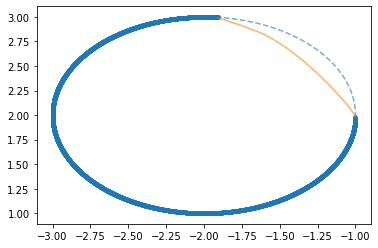# Increasing performance predicting curve chunk

Hi there !
Im still pretty new to neural nets and pytorch and actually this is my first post here. I wanted to create a simple neural net that could train on a chunk of a parametric curve and then predict a missing part (see image below).

I created my Dataset object in the following way:

``````X = torch.arange(-np.pi, np.pi, .001)
Y = torch.stack((torch.cos(X+1)-2, torch.sin(X+1)+2))
``````
``````class DS(Dataset):
def __init__(self, x, y):
self.x = x
self.y = y

def __getitem__(self, index):
return self.x[index], self.y[index]

def __len__(self):
return len(self.x)
``````
``````test_offset = 2120
test_size = 1500

test = [X[test_offset:test_offset + test_size], Y.t()[test_offset:test_offset + test_size]]
train = [torch.cat((X[0:test_offset], X[test_offset + test_size:])), torch.cat((Y.t()[0:test_offset], Y.t()[test_offset + test_size:]))]

test_ds = DS(X[test_offset:test_offset + test_size], Y.t()[test_offset:test_offset + test_size])
train_ds = DS(torch.cat((X[0:test_offset], X[test_offset + test_size:])), torch.cat((Y.t()[0:test_offset], Y.t()[test_offset + test_size:])))
``````

And a straighforward nn as:

``````class fede_net(nn.Module):
def __init__(self, leaky_slope = 0.1):
super(fede_net, self).__init__()
self.l1 = nn.Linear(1,100)
self.l2 = nn.Linear(100,100)
self.l3 = nn.Linear(100,100)
self.l4 = nn.Linear(100,50)
self.l5 = nn.Linear(50,2)
self.slope = leaky_slope

def forward(self, x):
x = self.l1(x)
x = F.leaky_relu(x , negative_slope= self.slope)
x = self.l2(x)
x = F.leaky_relu(x , negative_slope=self.slope)
x = self.l3(x)
x = F.leaky_relu(x , negative_slope=self.slope)
x = self.l4(x)
x = F.leaky_relu(x , negative_slope=self.slope)
x = self.l5(x)
return x
``````

Finally i train a net and print the predictions

``````second_lr = False
epochs = 20
for i in range(epochs):
for x_sample, y_sample in train_dl:
pred = net(x_sample.reshape(-1,1))
loss = loss_fn(pred, y_sample)
loss.backward()
optimizer.step()

if (second_lr==False)&(loss < 1e-5).item():
second_lr=True
print("Changing learning rate at epoch %d" %i)
optimizer = optim.Adam(net.parameters(), lr = 0.0001)

if i % 4 == 0:
print(str(i) + "/" + str(epochs), end = "\r")
print(loss)
pred_tot = net(test.reshape(-1,1))
plt.plot(train[:,0],train[:,1])
plt.plot(test[:,0],test[:,1], linestyle = '--')
plt.plot(pred_tot.detach()[:,0],pred_tot.detach()[:,1], alpha = i/epochs)

print(str(i) + "/" + str(epochs), end = "\r")
plt.show()
``````

The network actually works and predicts the missing part of the curve as you can see in the imageThe thing is i tried changing the size of the net both vertically and horizontally but could not get much better results. Actually i think that the size im using right now should be an overkill for this problem but no. I also tried changing the activation function to a tanh but didnt see any improvement either.

Any ideas on what should i try? Any feedback would be great !
Thanks in advance !!how do you expect for network to draw an arc absent from the training set, without knowledge about geometry?

what you’re seeing is like nearest neighbour inference (from 1d inputs!), the nature of that curvature is different

Is there any way i could try in order to improve this?

sinusoid activation extrapolates perfectly:

``````class fede_net(nn.Module):
def __init__(self, leaky_slope = 0.1):
super(fede_net, self).__init__()

self.h1 = nn.Linear(1,2)
self.h2 = nn.Linear(2,2)

def forward(self, x):
x = self.h1(x)
x = torch.sin(x)
x = self.h2(x)
return x